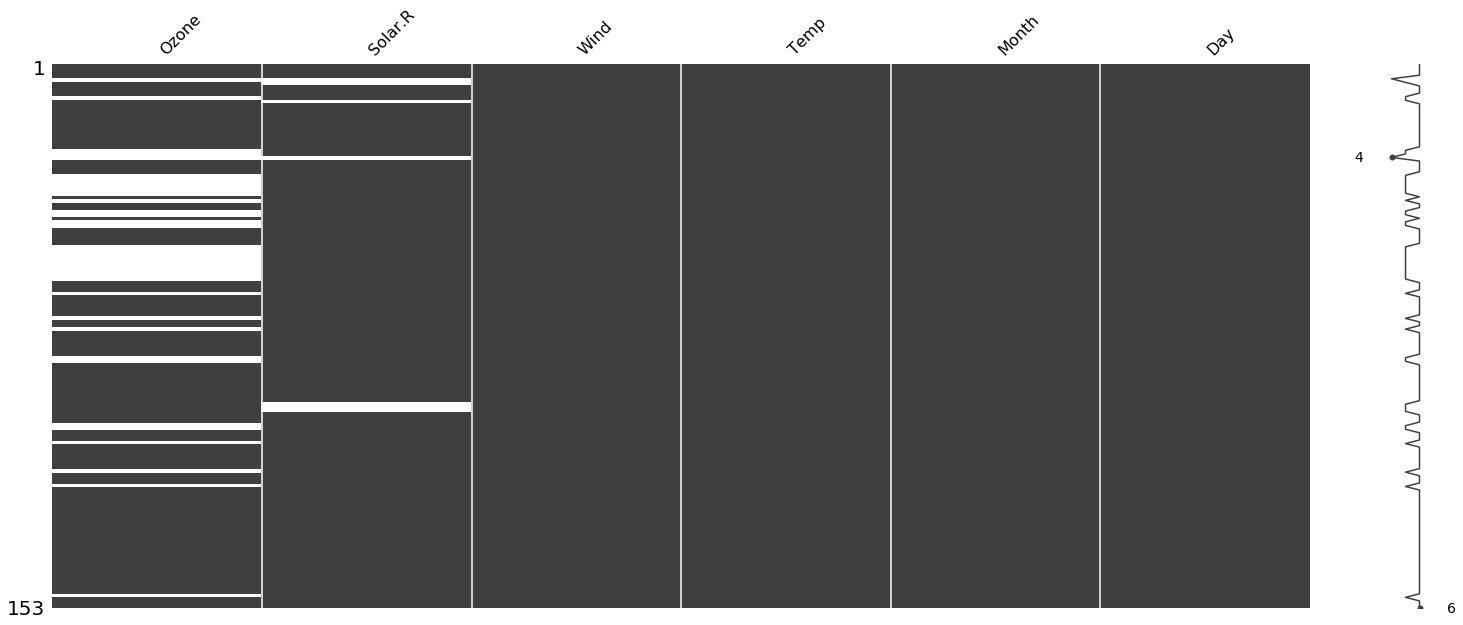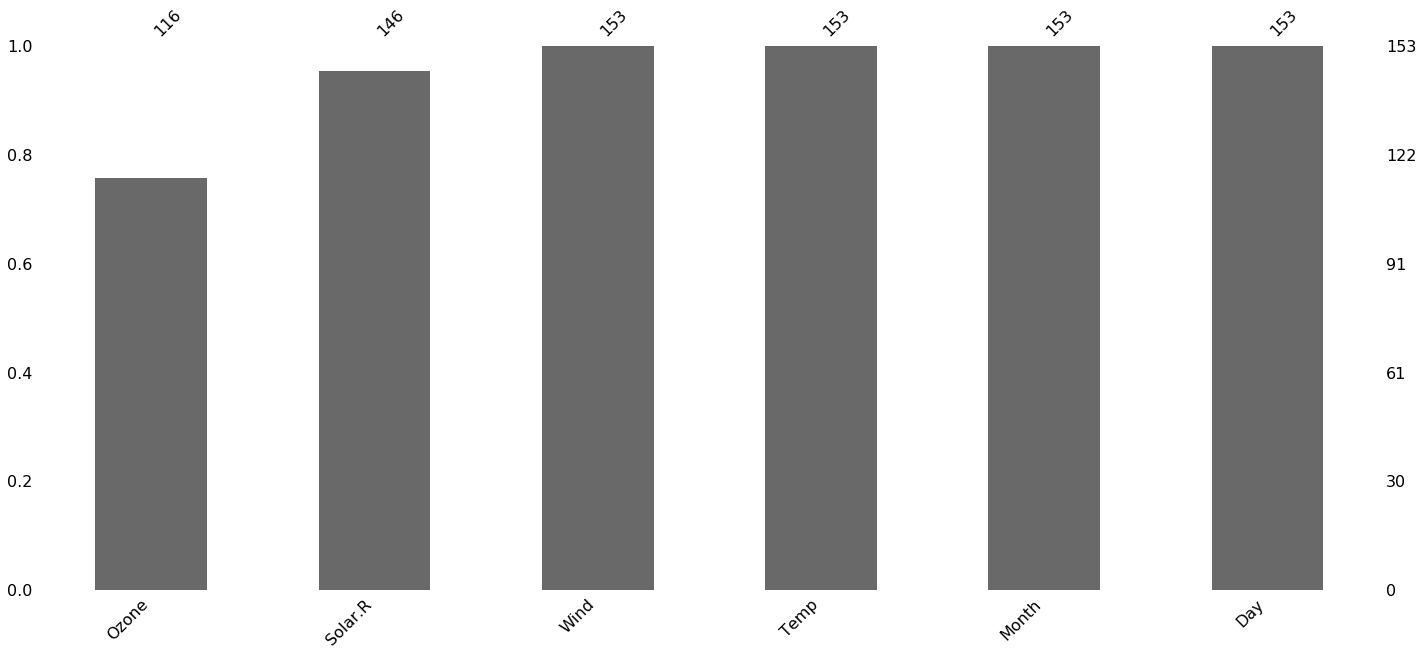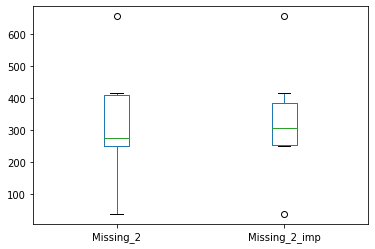## Missing Values

Missing values in a dataset is one of the most common problems and cleaning/preprocessing the data can affect the data analysis, ML model predictions. Missing values can come in many forms, e.g.

• Data are completely missing
• Values appear as N/A, Null, -, " . " etc. Data Analyst/Engineer/Scientist will have to code these values as missing
1. In some cases 0 may indicate a valid value while 0 may also indicate missing value. In such cases, domain knowledge helps to identify missing values.

## Missing Data Mechanism

Missing Completely At Random, MCAR: . There is no explanation as to why the data are missing and probability of missing is uniformly distributed. Missingness cannot be attributed to any other variables that are in the data or not included in the data. e.g. you have installed 100 sensors to measure temperature of a machine and few of the sensors ran out of battery or are just bad or not properly installed etc recorded no data for a brief amount of time. There is no systematic pattern to it and is just by luck. In this case, missingness is due to MCAR. Typically missingness by MCAR is few values. If missing data are large, then there is high probability that there are some variables causing the missingness.

Missing At Random, MAR: If we can attribute missing to other observed variables in the data, then the data are missing by MAR. For example, out of those 100 sensors, 25 were made by company A and usually the missingness is observed to be after 1000-1200 hours of installation when ambient temperature is between 90-110F. This indicates that the life of sensors by company A perhaps follow some weibull/exponential distribution dependent on duration and ambient temperature. Thus, there is high correlation between missing values and these factors (company, duartion and ambient temperature). This is more common and can be identified by exploratory data analysis. In general, it's good practice to assume the data are missing by MAR and try to identify the correlating factors.

Missing Not At Random, MNAR: If the data are missing neither by chance or by influence from observed data, then it is MNAR. This is the hardest mechanism to identify and address. This just means that missingness is caused by factors that are unknown and are external. Imagine some of the thermocouples in the example above are installed close to a vibrating part which vibrates excessively only when the load on the machine increases significantly, which causes the thermocouple wires to disconnect momentarily. Since there are multiple factors here that are not recorded, root cause is harder to pinpoint and can only be identified by experimentation and sensitivity (what-if analysis). While this may seem similar to MCAR, the difference is that MNRA has some probability of occurence (some pattern) while MCAR does not.

The mechanism of missingness informs which imputation strategy to use.

## Missing Value Quantification & Visualization

```import statsmodels.api as sm
import numpy as np

air = sm.datasets.get_rdataset("airquality").data
air.replace('NaN', np.nan, inplace=True)

print("Missing_Values_count: ", air.isna().sum())

print("Missing_Values_%: ", (air.isna().mean() * 100).round(1))
```
```Missing_Values_count:  Ozone      37
Solar.R     7
Wind        0
Temp        0
Month       0
Day         0
dtype: int64
Missing_Values_%:  Ozone      24.2
Solar.R     4.6
Wind        0.0
Temp        0.0
Month       0.0
Day         0.0
dtype: float64
```

missingno is a great library to visualize patterns in missing data

```import missingno as miss

miss.matrix(air);
```#### Barplot

```miss.bar(air);
```## Common Imputation Strategies

1. Listwise deletion : Delete all rows that have missing values. This is the easiest and often used strategy. This will will work only when the missingness is due to MCAR because the number of values are few (<1%) and are unlikely to affect the accuracy as it produces unbiased estimates under MCAR assumption. The drawbacks are :

• Size of observed data is reduced. This will affect non-linear models (e.g. tree based models) which benefit from more observations. Linear models (linear regression, logistic regression, SVM etc.) benefit from more features so may not be as much affacted.
• If the data are not MCAR, this will bias the population estimates.

In general if the missing data are few and can be attributed to MCAR, deletion is the most straightforward approach.

1. Single Value Imputation: Replace the missing data with population estimates such as mean, median, mode, percentile etc. This is by far the most used method of imputation. If the feature is symmatrically distributes, either mean or median will work but if it is skewed, median should be used to replace values. Drawbacks are:

• This is a problem if the data re MAR or MNAR as using a single value will definitely bias the estimates. Imagine in the example above, we replace the missing temperature with average temperature of 75F without giving regard to the ambient temperature. A non-linear model will capture this noise as a signal and will predict incorrect values.
• It reduces the variability in the feature, and thus predictive power of that feature
• ALWAYS split the data first, calculate the mean/median etc for the training set and use the SAME mean/median for the test/evaluation set. Apply scaling and other transformations after imputing.
1. Forward/Backward Fill/Interpolation: This is typically used in time series analysis when there is high autocorrelation in the data, i.e values are correlated to its past/future. We would either carry forward the last value to fill the missing value or calculate moving average (centrak or expanding window) and then fill the value. The main drawback is that it doesnt work with highly non-linear features or when the data have no autocorrelation. Interpolation will work by interpolating over the data. We can choose linear, quadratic, cubic etc. This will require some domain knowledge to choose the right interpolation method.
1. Indicator Variable: This is typically used for missing categorical data. This can be thought of as feature engineering. Replace the missing category with a category (e.g. "missing" or "N/A" etc.) and add another dummy column that indicates the category was missing. The ML algorithm will treat it as a feature and if you have large enough dataset, will learn the missingness pattern. This will work best for non-linear algorithms.
1. MICE ( Multiple Imputation By Chained Equation): MICE does multivariate regression analysis that is multiple times to converge at values. This is a very useful technique, especially when the data are missing by MAR. It is a good practice to compare summary statistics of the missing variable before and after applying MICE. MICE is a very robust imputation method.
1. KNN Imputation: K-nearest Neighbor can be used to find samples in the training set that are closest to the missing values and average the nearby points to predict the missing value. The purpose of imputation is not only to find possible true value but also to keep the original data structure. It's been shown that kNN imputation achieves both very efficiently and is a very effective technique Ref.

While sklearn/statsmodels/pandas offers all of the above imputation techniques, Azure ML does not. Azure ML Studio Classic had MICE and Probabilistic PCA but they are absent from the Designer. I discuss some of the above techniques in the video below.

To demonstrate some of the options avialable, I will use the following example table I created. This dataset

Index Missing_1 Missing_2 Missing_6 Misisng_8 Missing_Cat1 Missing_Ca2 Missing_2-2
0 1 320.0 249.0 NaN 224.0 Gold Gold 26
1 2 613.0 251.0 NaN NaN Blue Blue 74
2 3 260.0 294.0 590.0 NaN Silver Silver 174
3 4 419.0 NaN NaN NaN Red Red 0
4 5 485.0 415.0 386.0 NaN Blue Blue 488
5 6 NaN 655.0 NaN NaN Silver NaN 435
6 7 61.0 37.0 NaN NaN Red Red 588
7 8 41.0 NaN NaN NaN Yellow Yellow -
8 9 607.0 254.0 369.0 NaN Blue Blue 363
9 10 423.0 407.0 80.0 495.0 Blue Blue 512

Update: I forgot to show in the video how single value imputation reduces variability. Consider the column Missing_2. With missing values, the standard deviation of the column is 178. But if we replace the 2 missing values with the mean of the column, 320, the standard deviation is 157. The more values we replace, the lower the variability. If the variance reduces significantly, it will not be an effective predictor of the outcome. Note that the mean remains the same after imputation.

```print("Mean :,",data["Missing_2"].mean())
print("Std :", data["Missing_2"].std())

data["Missing_2_imp"] = data["Missing_2"].replace(np.nan,data["Missing_2"].mean())
```
```Mean :, 320.25
Std : 178.7821899087586
```
```print("Mean_after:",data["Missing_2_imp"].mean())
print("Std_after:",data["Missing_2_imp"].std())
```
```Mean_after: 320.25
Std_after: 157.67107111536558
```
```data[["Missing_2","Missing_2_imp"]].plot.box();
```